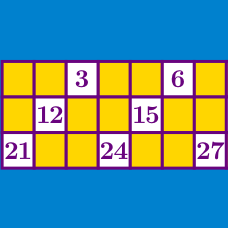Number Theory

# Application of Divisibility Rules

Find the digit $$N$$ such that the three digit integer $$\overline{ 5 7 N}$$ is a multiple of 9.

How many digits $$N$$ are there such that the six-digit integer $$\overline{1 2 3 3 N 2}$$ is a multiple of 8?

Find the digit $$N$$ such that the five-digit number $$\overline{N878N}$$ is divisible by 9.

How many three-digit numbers are not divisible by $$5,$$ have digits that sum to $$15,$$ and have the first digit equal to the third digit?

How many 5 digit positive integers are multiples of 11, and have digits which sum to 43?

×